# Digital Control of dc motor question advice

#### Saviour Muscat

Joined Sep 19, 2014
175
Dear helpers,

This is one of the last units of my part-time engineering course, hope to conclude it well with your kind help.
Currently, I have a digital control scenario question of a cascaded control of a position/velocity of a dc motor. I'm trying to tackle questions one by one and advise me if I'm in the right way. Attached in fig1 the coursework question, fig2 the tentative solution block diagram of B3 a).
For GP1 and GP2,

For GP2=1/s

For GP1, I did this derivation illustrated on fig3 referred to block diagram fig 2, kindly advice

GP2=0.01/(5.00625*10^-3s^2+0.060075s+0.100125)

Thank you

#### MrAl

Joined Jun 17, 2014
9,357
Dear helpers,

This is one of the last units of my part-time engineering course, hope to conclude it well with your kind help.
Currently, I have a digital control scenario question of a cascaded control of a position/velocity of a dc motor. I'm trying to tackle questions one by one and advise me if I'm in the right way. Attached in fig1 the coursework question, fig2 the tentative solution block diagram of B3 a).
For GP1 and GP2,

For GP2=1/s

For GP1, I did this derivation illustrated on fig3 referred to block diagram fig 2, kindly advice

GP2=0.01/(5.00625*10^-3s^2+0.060075s+0.100125)

Thank you
Hello,

Your block diagram looks correct and your resulting equation looks correct.
After plugging in the given constants i find only minor difference in the solution you got and mine.
Mine is:
0.01/(0.00500625*s^2+0.060075015625*s+0.100125)
Yours was:
0.01/(5.00625*10^-3s^2+0.060075s+0.100125)

and so i am assuming that you rounded that central constant, the one multiplied by 's'.
I am also assuming you do not have to include the disturbance torque.

•Saviour Muscat

#### Saviour Muscat

Joined Sep 19, 2014
175
Hello,

Your block diagram looks correct and your resulting equation looks correct.
After plugging in the given constants i find only minor difference in the solution you got and mine.
Mine is:
0.01/(0.00500625*s^2+0.060075015625*s+0.100125)
Yours was:
0.01/(5.00625*10^-3s^2+0.060075s+0.100125)

and so i am assuming that you rounded that central constant, the one multiplied by 's'.
I am also assuming you do not have to include the disturbance torque.
Dear MrAl,

I truly appreciate your help, just saw I had some rounding in the equation.

Thank you very much,

#### MrAl

Joined Jun 17, 2014
9,357
Dear MrAl,

I truly appreciate your help, just saw I had some rounding in the equation.

Thank you very much,
Hi,

You are welcome.
Also, it may be ok to round, but when i see that they used higher precision to quote the physical constants i hesitated to round because it may be the tiny portions they wanted to see correct. In real life we would probably round like you did though, but then gain i doubt we would be able to make the measurements of the physical constants as well as they seem to have doneMore than likely they are made up constants just for the exercise (note the extreme precision of the friction and inductance values which would probably be impossible to measure that accurately).

•nsaspook

#### Saviour Muscat

Joined Sep 19, 2014
175
Hello,

Thank you for your support

Sorry for the inconvenience done, due personal reasons and work issues I couldn't continue to tackle this assignment without delay.

Now I am trying to tackle question b of the discrete Open loop TF(GH) of the velocity which I am little bit confused about the sampler at the feedback. I don't know if my working is correct but I tried to do it. Could someone verify my tentative solution please.

Thanks

Saviour

#### Saviour Muscat

Joined Sep 19, 2014
175
Hello,

Thank you for your support

Sorry for the inconvenience done, due personal reasons and work issues I couldn't continue to tackle this assignment without delay.

Now I am trying to tackle question b of the discrete Open loop TF(GH) of the velocity which I am little bit confused about the sampler at the feedback. I don't know if my working is correct but I tried to do it. Could someone verify my tentative solution please.

Thanks

Saviour

TY
SM

#### MrAl

Joined Jun 17, 2014
9,357

TY
SM
Hi,

Since no one else replied yet i'll try to take a look at this a bit later today.

#### Saviour Muscat

Joined Sep 19, 2014
175

#### Saviour Muscat

Joined Sep 19, 2014
175

#### MrAl

Joined Jun 17, 2014
9,357
Gentile reminder

TY

Saviour
How did you know i was a gentile? Ha ha, i bet you meant "gentle"Check the definitions for a laugh.

OK you did pretty good but it looks like when you formed the common denominator you didnt distribute it properly.
Check the attachment, blue star  looks correct (with rounding accepted that is) but red star  does not. Go over that part see what you think.

Side note:
It is faster for someone to check your work if you can also provide a pure text version of your results. That is because typing in long numbers to check results using math software takes much time and care. With a pure text version we can just copy and paste and arrive at a solution check in minutes or even just seconds.
Consider typing this number all by hand into your math software:
pi^2=9.869604401089358618834490999876151135313699407240790626413349374285977301132874762146178862115173871455167
Is that correct to the specified number of digits?
You can copy and paste that but if it was an image (like png, gif, jpg, etc.) then you would have to spend time typing it in unless you had good OCR software that could read it without errors.

Last edited:

#### Saviour Muscat

Joined Sep 19, 2014
175
Hello, I am back,

I did step by step for your kind help
K2*(1-z^-1)(0.01/(0.00500625s^3+0.060075s^2+0.100125s)) , ZOH =(1-e^-sT)/s

Solve using partial fractions-0.124844/(s + 2) + 0.0249688/(s + 10) + 0.0998752/s

using z-Transform table gives

0.0998752/(z-1)-0.124844/(z-e^-2*0.1)+0.0249688/(z-e^-10*0.1)

z*(0.0998752/(-1 + 1 z) - 0.124844/(-0.818731 + 1 z) + 0.0249688/(-0.367879 + 1 z))

(0.0998752/(-1 + 1 z) - 0.124844/(-0.818731 + 1 z) + 0.0249688/(-0.367879 + 1 z))*((z*K_2*(z-1))/(z))

finally Open Loop TF function is:

(K_2)*(0.00684704 z + 0.00459705)/(z^2 - 1.18661 z + 0.301194)

Kindly could you comment the above result if is it correct|?

And I did the same with Matlab and gives the same result, I suppose is it correct, kindly comment also below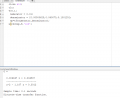#### Saviour Muscat

Joined Sep 19, 2014
175
Hi,

I did also the discrete closed loop TF, X=K_2, using G/(1+GH) for velocity

(X (0.671392 + z))/(43.9889 + X (0.671392 + z) + z (-173.303 + 146.049 z))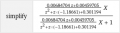Open loop for positional system is GH, D(z)*(1/s)*(Discrete Closed LOOP velocity tf), D(z)*(z/(z-1))*(X (0.671392 + z))/(43.9889 + X (0.671392 + z) + z (-173.303 + 146.049 z))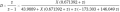Closed loop for positional discrete TF G/(1+GH)=

(D*(z/(z-1))*(X (0.671392 + z))/(43.9889 + X (0.671392 + z) + z (-173.303 + 146.049 z)))/(D*(z/(z-1))*(X (0.671392 + z))/(43.9889 + X (0.671392 + z) + z (-173.303 + 146.049 z))+1)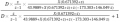Again TY

Saviour

#### MrAl

Joined Jun 17, 2014
9,357
Hello, I am back,

I did step by step for your kind help
K2*(1-z^-1)(0.01/(0.00500625s^3+0.060075s^2+0.100125s)) , ZOH =(1-e^-sT)/s

Solve using partial fractions-0.124844/(s + 2) + 0.0249688/(s + 10) + 0.0998752/s

using z-Transform table gives

0.0998752/(z-1)-0.124844/(z-e^-2*0.1)+0.0249688/(z-e^-10*0.1)

z*(0.0998752/(-1 + 1 z) - 0.124844/(-0.818731 + 1 z) + 0.0249688/(-0.367879 + 1 z))

(0.0998752/(-1 + 1 z) - 0.124844/(-0.818731 + 1 z) + 0.0249688/(-0.367879 + 1 z))*((z*K_2*(z-1))/(z))

finally Open Loop TF function is:

(K_2)*(0.00684704 z + 0.00459705)/(z^2 - 1.18661 z + 0.301194)

Kindly could you comment the above result if is it correct|?

And I did the same with Matlab and gives the same result, I suppose is it correct, kindly comment also below

View attachment 276126
The only part wrong was the part that i mentioned previously.
You can check your result with mine in the attachment. It's the same except for rounding.
I also plotted the step response of that transfer function just to see what it would look like.

#### Saviour Muscat

Joined Sep 19, 2014
175
Hello,

Your intuition to find my mistake was very useful, thank you,

I tried to answer question B4 to find the steady state error

Wo= (K_2)*(0.00684704 z + 0.00459705)/(z^2 - 1.18661 z + 0.301194) open loop tf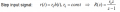for step input =h(t)=z/(z-1), r_o=1

steady state error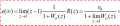1/((K_2)*(0.00684704 z + 0.00459705)/(z^2 - 1.18661 z + 0.301194)+1), where K_2=16.513 for critical damping found from sisotool

Error=as z approaches 1 =0.377467

Kindly, could you verify my answer, please?

#### MrAl

Joined Jun 17, 2014
9,357
Hello,

Your intuition to find my mistake was very useful, thank you,

I tried to answer question B4 to find the steady state error

Wo= (K_2)*(0.00684704 z + 0.00459705)/(z^2 - 1.18661 z + 0.301194) open loop tf

View attachment 276171

for step input =h(t)=z/(z-1), r_o=1

steady state error View attachment 276169

1/((K_2)*(0.00684704 z + 0.00459705)/(z^2 - 1.18661 z + 0.301194)+1), where K_2=16.513 for critical damping found from sisotool

Error=as z approaches 1 =0.377467

Kindly, could you verify my answer, please?
Ok i'll take a look at this, but before that i just wanted to mention that the step response i provided does not include the constant factor K2 i dont think you gave that before now? If you did i missed it, but doesnt matter that much just multiply everything by the new value of K2 you provided. I'll also double check that step response plot to make sure it is right or will correct it as that tells us a lot about the response.

#### Saviour Muscat

Joined Sep 19, 2014
175
TY MrAl,

I did your recommendations and corrected some mistakes

also I did by mistake wrong damping ratio i.e zeta=1 and k2 is found to be 5.955 by sisotool
1/((K_2)*(0.00684704 z + 0.00459705)/(z^2 - 1.18661 z + 0.301194)+1)
and expression for the error is 1/(1+0.099875*k2)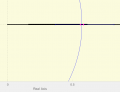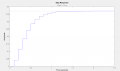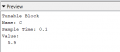#### MrAl

Joined Jun 17, 2014
9,357
TY MrAl,

I did your recommendations and corrected some mistakes

also I did by mistake wrong damping ratio i.e zeta=1 and k2 is found to be 5.955 by sisotool
1/((K_2)*(0.00684704 z + 0.00459705)/(z^2 - 1.18661 z + 0.301194)+1)
and expression for the error is 1/(1+0.099875*k2)

View attachment 276185View attachment 276186

View attachment 276187
Hello,

Oh i see you corrected another slight problem where you multiplied by z/(z-1) where you did not need that. That's good too.

So you want to change the value of K2 to 5.955 now? Are you sure because that will result in a much smoother response but longer rise time. It will get rid of any overshoot however, if that is what you were looking for.
Also, this will be valid for a step response but probably not for a higher order polynomial input. That may not be an issue here though because a lot of this was given before you started.

Ok i'll verify your results next and provide a step response to verify with yours. That step response doesnt look right but i'll check it more carefully next.

#### MrAl

Joined Jun 17, 2014
9,357
TY MrAl,

I did your recommendations and corrected some mistakes

also I did by mistake wrong damping ratio i.e zeta=1 and k2 is found to be 5.955 by sisotool
1/((K_2)*(0.00684704 z + 0.00459705)/(z^2 - 1.18661 z + 0.301194)+1)
and expression for the error is 1/(1+0.099875*k2)

View attachment 276185View attachment 276186

View attachment 276187

Hello again,

Ok i verified your step response but i think you need to take another look at this and think about whether or not it is a good idea to reduce the gain to 5 or 6 down from around 16 or 17. That's a big change.
The reason we would aim for high gain is to get a small steady state error. The idea being what good is the control system if the steady state error is too high, and especially not if the SS error is as high as the output itself, i think that would be a pretty nutty system. IF the SS error is higher than the output, that's really nuttyYour math is correct though, now you just need to look a the steady state error a little better. The goal is to get it down as much as possible while maintaining a smooth enough output response such that it does not ring too much before it settles down, so you also have to think about how much ringing is acceptable and how much is not acceptable, and how much steady state error is acceptable and how much is not.
In the end it will be a tradeoff, but really even the original 38 percent SS error does not sound very good. Again the math is right, just the design goal does not sound like it is being achieved yet although i am not completely sure what you are after yet.

See if you can plot the steady state error along with your output response plot. That should reveal more about this.
Here is the step response plot i got. It is similar to yours with the new gain, but i plotted the step response with the old gain of 16.513 to show how much error there is along with the response itself.
The horizontal green line is the idealized response which would be the response for a perfect system which is not really attainable just there for comparison.